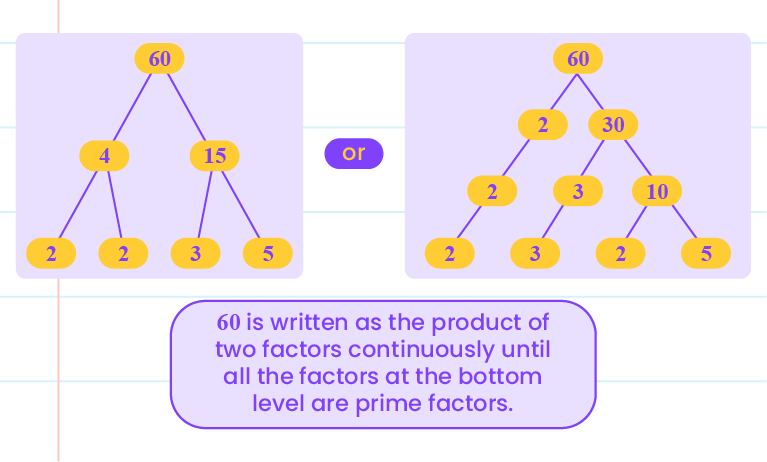## Factors, Prime Factors and Highest Common Factor (HCF)

 2.1 Factors, Prime Factors and Highest Common Factor (HCF)

 Factors A number that divides another number completely. The factors of $$12$$ are $$1, 2,3,4,6$$ and $$12$$.

 Example Is $$12$$ the factor of $$36$$? $$36\div 12=3$$ Thus, $$12$$ is the factor of $$36$$.

 Prime Factor Factor that is a prime number.

 Example The factors of $$18$$ are $$1, 2,3,6,9$$ and $$18$$. Between these factors, $$2$$ and $$3$$ are prime numbers. Thus, the prime factors of $$18$$ are $$2$$ and $$3$$.

 Solution Methods Repeated division: Express $$60$$ in the form of prime factorisation. Perform division repeatedly by dividing with the smallest prime number. Division is continued until the quotient is $$1$$. $$\begin{array}{c} 2\\2\\3\\5 \\\phantom{-} \end{array} \begin{array}{|c} \quad60\quad\\ \hline \quad30\quad\\ \hline \quad15\quad\\ \hline \quad5\quad\\ \hline \quad1\quad\\ \end{array} \begin{array}{c}\end{array}\\\\$$ Thus,  $$60=2\times2\times3\times5$$. Factor trees: Express $$60$$ in the form of prime factorisation.Thus,  $$60=2\times2\times3\times5$$.

 Common Factor A number that is a factor of a few other numbers.

 Example Determine whether $$6$$ is a common factor of $$24$$ and $$36$$. $$24\div6=4 \\36\div6=6$$ $$24$$ and $$36$$ can be divided completely by $$6$$. Thus, $$6$$ is a common factor of $$24$$ and $$36$$.

 Highest Common Factor (HCF) The greatest number among the common factors.

Example

(i) Determine the highest common factor of $$18$$ and $$24$$.

Listing the common factors:

Factors of $$18 : 1 , 2 , 3 , 6 , 9, 18$$

Factors of $$24 : 1 , 2 , 3 , 4 , 6 , 8, 12, 24$$

So, the common factors of $$18$$ and $$24$$ is $$1, 2, 3$$ and $$6$$.

Thus, HCF is $$6$$.

(ii) Determine the highest common factor of $$30,60$$ and $$72$$.

Repeated division:

$$\begin{array}{c} 2\\2\\3 \\\phantom{-} \end{array} \begin{array}{|c} \quad36,\,60,\,72\quad\\ \hline \quad18,\,30,\,36\quad\\ \hline \quad9,\,15,\,18\quad\\ \hline \quad3,\,5,\,6\quad\\ \end{array} \begin{array}{c}\end{array}\\\\$$

Thus, HCF of $$36, 60$$ and $$72$$ is

$$2\times2\times3 = 12$$.

(iii) Determine the highest common factor of $$48,64$$ and $$80$$.

Prime factorisation:

$$48 = 2\times2\times 2\times2\times3$$

$$64 = 2 × 2 × 2 × 2 × 2 × 2$$

$$80 = 2\times2\times 2\times 2\times5$$

Thus, HCF is

$$2 × 2 × 2 × 2 = 16$$.